# ZeroDivisionError: float division by zero in Python [Fixed]Last updated: Feb 16, 2023
6 min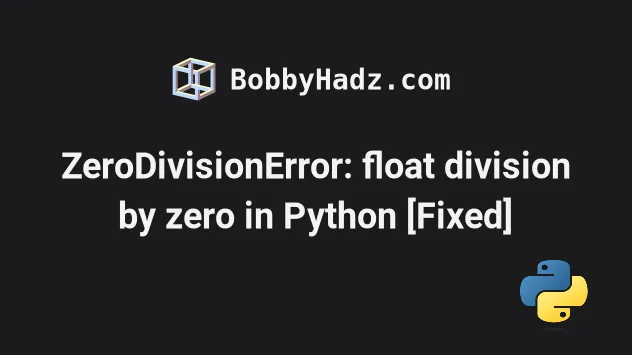## #ZeroDivisionError: float division by zero in Python

The Python "ZeroDivisionError: float division by zero" occurs when we try to divide a floating-point number by `0`.

To solve the error, use an `if` statement to check if the number you are dividing by is not zero, or handle the error in a `try/except` block.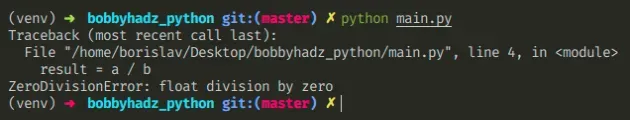Here is an example of how the error occurs.

main.py
```Copied!```a = 15.0
b = 0

# ⛔️ ZeroDivisionError: float division by zero
result = a / b
``````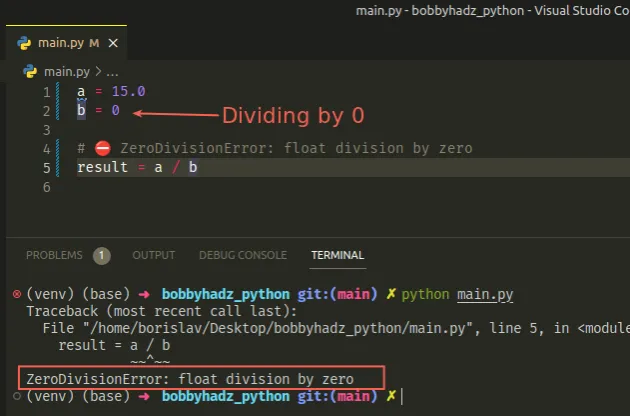It's unclear what value is expected when we divide by `0`, so Python throws an error.

When we divide a number by `0`, the result tends towards infinity.

## #Checking if the value we are dividing by is not 0

One way to solve the error is to check if the value we are dividing by is not `0`.

main.py
```Copied!```a = 15.0
b = 0

if b != 0:
result = a / b
else:
result = 0

print(result)  # 👉️ 0
``````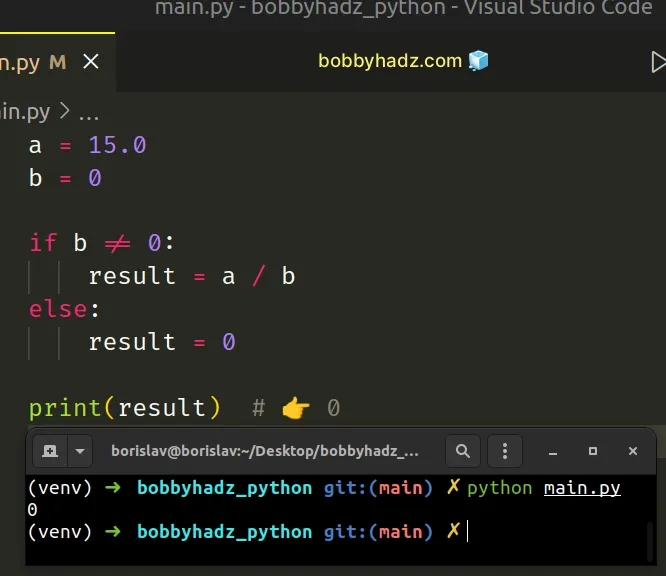We check if the `b` variable doesn't store a `0` value and if it doesn't, we divide `a` by `b`.

Otherwise, we set the `result` variable to `0`. Note that this could be any other value that suits your use case.

If setting the `result` variable to `0` if `b` is equal to `0` suits your use case, you can shorten this to a single line.

main.py
```Copied!```a = 15.0
b = 0

result = b and a / b

print(result)  # 👉️ 0
``````

The expression `x and y` first evaluates `x`, and if `x` is falsy, its value is returned, otherwise, `y` is returned.

Since `0` is a falsy value, it gets returned if the `b` variable in the example stores a `0` value, otherwise, the result of dividing `a` by `b` is returned.

## #Using a `try/except` statement to handle the error

Alternatively, you can use a `try/except` statement.

main.py
```Copied!```a = 15.0
b = 0

try:
result = a / b
except ZeroDivisionError:
result = 0

print(result)  # 👉️ 0
``````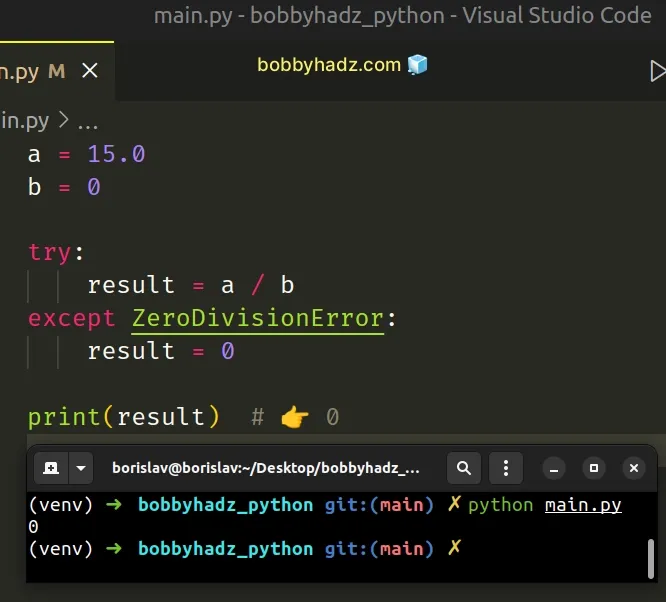We try to divide `a` by `b` and if we get a `ZeroDivisionError`, the `except` block sets the `result` variable to `0`.

## #Figure out where the variable got set to `0`

The best way to solve the error is to figure out where the variable gets assigned a `0` and check whether that's the expected behavior.

Here are some common ways you might get a zero value unexpectedly.

main.py
```Copied!```print(int())  # 👉️ 0
print(int(0.9))  # 👉️ 0
``````

You might also get a zero value if you multiply any number by `0`.

main.py
```Copied!```num_1 = 5

num_2 = num_1 * 0

print(num_2) # 👉️ 0
``````

Make sure you haven't assigned the result of multiplying a number by `0` to a variable.

## #Handling a number from user input with a `try/except` statement

If you need to take a number from user input, use a `try/except` statement to handle the potential `ZeroDivisionError`.

main.py
```Copied!```num_1 = 15.0

try:
num_2 = int(input('Enter a number: '))
result = num_1 / num_2

print(f'The result of the division is: {result}')
except (ZeroDivisionError, ValueError):
print('Specify a positive integer value')
``````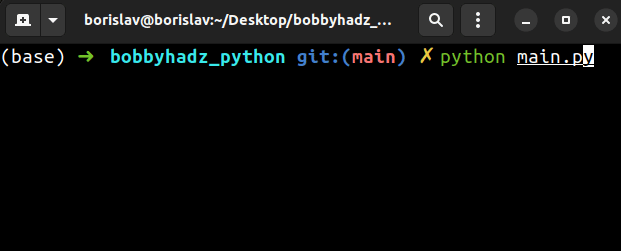If the user passes an invalid integer, a `ValueError` is raised and is then handled by the `except` block.

Similarly, if the user enters `0`, a `ZeroDivisionError` is raised and is handled by the `except` block.

Otherwise, the result of the division gets printed in the `try` block.

## #ZeroDivisionError: integer modulo by zero in Python

The Python "ZeroDivisionError: integer division or modulo by zero" occurs when we use the modulo `%` operator with an integer and a zero.

To solve the error, figure out where the `0` comes from and correct the assignment.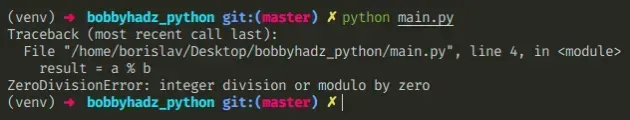Here is an example of how the error occurs.

main.py
```Copied!```a = 6
b = 0

# ⛔️ ZeroDivisionError: integer division or modulo by zero
# ZeroDivisionError: integer modulo by zero
result = a % b
``````

We tried using the modulo `%` operator with a zero.

It's unclear what value is expected when we divide by `0`, so Python throws an error.

When we divide a number by `0`, the result tends towards infinity.

## #Figuring out where the variable got assigned a zero value

The best way to solve the error is to figure out where the `0` value comes from and correct the assignment.

Here are some unexpected sources of `0`.

main.py
```Copied!```import random

print(int())  # 👉️ 0
print(int(0.9))  # 👉️ 0

print(random.randint(0, 10))  # 👉️ 0

print(list(range(0, 5)))  # 👉️ [0, 1, 2, 3, 4]
``````

If you use the `random.randint()` method or the `range()` class, make sure to start from `1`, and not from `0`.

main.py
```Copied!```import random

print(random.randint(1, 10))  # 👉️ 4

# 👇️ [1, 2, 3, 4]
print(list(range(1, 5)))
``````

## #Check if the value is not `0` before using modulo

You can also conditionally check if the variable doesn't store a `0` value before using the modulo operator.

main.py
```Copied!```a = 6
b = 0

if b != 0:
result = a % b
else:
# 👇️ this runs
print('b is equal to 0')
``````

The `if` statement checks if the `b` variable doesn't store a `0` value before using the modulo `%` operator.

## #Using a `try/except` statement to handle the error

Alternatively, you can use a try/except statement.

main.py
```Copied!```a = 6
b = 0

try:
result = a % b
print(result)
except ZeroDivisionError:
pass
``````

We use the modulo operator and if we get a `ZeroDivisionError`, the `except` block is run.

You can set the `result` variable to a value that suits your use case in the `except` block or simply pass.

The modulo (%) operator returns the remainder from the division of the first value by the second.

main.py
```Copied!```print(10 % 2)  # 👉️ 0
print(10 % 4)  # 👉️ 2
``````

If the value on the right-hand side is zero, the operator raises a `ZeroDivisionError` exception.

The left and right-hand side values may also be floating point numbers.

If the left-hand side value is a float and the right-hand side value is `0`, you would get a "ZeroDivisionError: float modulo" error.

main.py
```Copied!```# ⛔️ ZeroDivisionError: float modulo
print(10.5 % 0)  # 👉️ 0
``````

## #ZeroDivisionError: division by zero in Python

The Python "ZeroDivisionError: division by zero" occurs when we try to divide a number by `0`.

To solve the error, use an `if` statement to check if the number you are dividing by is not zero, or handle the error in a `try/except` block.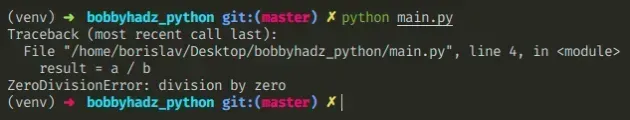Here is an example of how the error occurs.

main.py
```Copied!```a = 5
b = 0

# ⛔️ ZeroDivisionError: division by zero
result = a / b
``````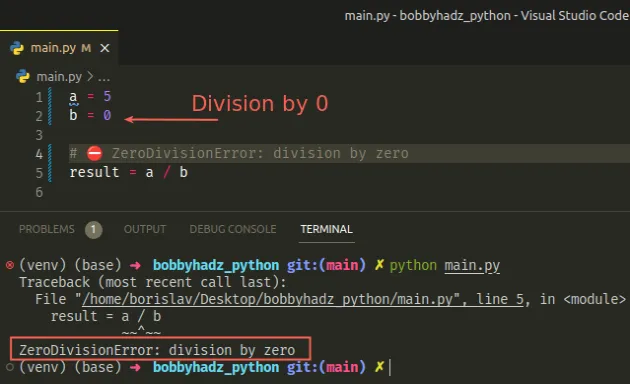It's unclear what value is expected when we divide by `0`, so Python throws an error.

When we divide a number by `0`, the result tends towards infinity.

## #Checking if the value you are dividing by is not zero

One way to solve the error is to check if the value we are dividing by is not `0`.

main.py
```Copied!```a = 5
b = 0

if b != 0:
result = a / b
else:
result = 0

print(result)  # 👉️ 0
``````

We check if the `b` variable doesn't store a `0` value and if it doesn't, we divide `a` by `b`.

Otherwise, we set the `result` variable to `0`. Note that this could be any other value that suits your use case.

If setting the `result` variable to `0`, if `b` is equal to `0` suits your use case, you can shorten this to a single line.

main.py
```Copied!```a = 5
b = 0

result = b and a / b

print(result)  # 👉️ 0
``````

The expression `x and y` first evaluates `x`, and if `x` is falsy, its value is returned, otherwise, `y` is returned.

Since `0` is a falsy value, it gets returned if the `b` variable in the example stores a `0` value, otherwise the result of dividing `a` by `b` is returned.

## #Using a `try/except` statement to handle the error

Alternatively, you can use a `try/except` statement.

main.py
```Copied!```a = 5
b = 0

try:
result = a / b
except ZeroDivisionError:
result = 0

print(result)  # 👉️ 0
``````

The `try/except` block is known as "asking for forgiveness, rather than permission".

We try to divide `a` by `b` and if we get a `ZeroDivisionError`, the `except` block sets the `result` variable to `0`.

The best way to solve the error is to figure out where the variable gets assigned a `0` and check whether that's the expected behavior.

Here are some common ways you might get a zero value unexpectedly.

main.py
```Copied!```print(int())  # 👉️ 0
print(int(0.9))  # 👉️ 0
``````

You might also get a zero value by multiplying a number by `0`.

I've also written an article on how to check if a number is divisible by another number.